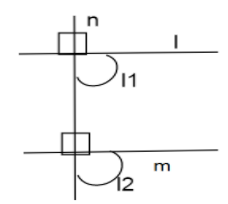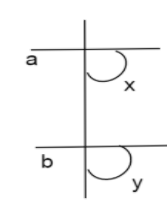QuestionAnswers

# Two lines $I$ and $m$ are perpendicular to the same line $n$ . Are $I$ and $m$ perpendicular to each other? (State whether the given statement is true or false.)Verified
92.4k+ views
Hint: Here we will use the drawing for this question. Using this drawing we will find out the answer if it is true or false, we will explain the question why it is true why it is false. Now we will find if it is perpendicular or it is the same line.

Complete step-by-step solution:
Here first we will draw an angle for given question then only we will find the solutionHere $\left| \!{\underline {\, {l1} \,}} \right. = {90^ \circ },\left| \!{\underline {\, {l2} \,}} \right. = {90^ \circ }$
Therefore here $l\parallel m$
$l1$ and $l2$ are not parallel to each other, here corresponding angles are equal.Here also$\left| \!{\underline {\, x \,}} \right. = \left| \!{\underline {\, y \,}} \right.$
Here $a\parallel b$
$a,b$ are parallel to each other.

The given statement is false.

Note: When two rays (part of a straight line) intersect each other in the same plane, they form an angle. The point of intersection is called a vertex. Also having corresponding angles and interior alternate angles.
When a transversal intersects two parallel lines, the corresponding angles are equal. The vertically opposite angles are equal. The alternate interior angles are equal. A line that has one definite endpoint is called a ray and extends endlessly in one direction. A ray is named after the endpoint and another point on the ray.